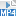## Content

### Applying the binomial theorem

In this section, we give some examples of applying the binomial theorem.

#### Example

Expand $$(2x+3)^4$$.

#### Solution

Using the binomial theorem:

$(a+b)^4 =a^4+4a^3b+6a^2b^2 +4ab^3+ b^4.$

Let $$a =2x$$ and $$b=3$$. Then

\begin{align*} (2x+3)^4 &= (2x)^4+ 4(2x)^3\times3+6(2x)^2\times3^2+ 4(2x)\times3^3+ 3^4\\ &=16x^4+ 96x^3+216x^2+216x+ 81. \end{align*}

#### Example

Expand $$(1-x)^{10}$$.

#### Solution

Using the binomial theorem:

\begin{align*} (a+b)^n &= \sum_{r=0}^{n}\dbinom{n}{r}a^{n-r}b^r. \end{align*}

Let $$a=1$$, $$b=-x$$ and $$n = 10$$. Then

\begin{align*} &(1-x)^{10} = \sum_{r=0}^{10}\dbinom{10}{r}(-x)^r\\ &= 1-10x+45x^2-120x^3+210x^4-252x^5+210x^6-120x^7+45x^8-10x^9+x^{10}. \end{align*}
##### Exercise 5

Expand

1. $$(2x-3y)^4$$
2. $$\Big(x-\dfrac{2}{x}\Big)^4$$.

Screencast of exercise 5#### The general term in the binomial expansion

The general term in the expansion of $$(a+b)^n$$ is

$\dbinom{n}{r}a^{n-r}b^r, \qquad\text{where}\quad 0 \leq r\leq n.$

#### Example

For the expansion of $$(x-3z^2)^5$$, find the general term and find the coefficient of $$x^3z^4$$.

#### Solution

The general term is

$\dbinom{5}{r}x^{5-r}(-3z^2)^r, \qquad\text{where}\quad 0 \leq r\leq 5.$

To find the coefficient of $$x^3z^4$$, we want $$5-r=3$$ and $$r=2$$.

Thus the coefficient of $$x^3z^4$$ is $$\dbinom{5}{2}(-3)^2 = 90$$.

##### Exercise 6

Consider the expression $$(a-2b^3)^7$$. How many terms are there in the expansion? Find a formula for the general term.

#### Example

Find the constant term in the expansion of $$\Big(x^2 + \dfrac{1}{x^2}\Big)^6$$.

#### Solution

The general term is

\begin{align*} \dbinom{6}{r}\big(x^2\big)^{6-r}\Big(\dfrac{1}{x^2}\Big)^r &= \dbinom{6}{r}x^{12-2r}\Big(\dfrac{1}{x^{2r}}\Big)\\ &=\dbinom{6}{r}x^{12-4r}. \end{align*}

This term will be a constant when $$12-4r=0$$, that is, when $$r=3$$.

Hence the constant term in the expansion of $$\Big(x^2 + \dfrac{1}{x^2}\Big)^6$$ is $$\dbinom{6}{3}= 20$$.

##### Exercise 7

Find the constant term in the expansion of $$\Big(x + \dfrac{1}{x^2}\Big)^6$$.

Screencast of exercise 7#### Example

Find the coefficient of $$x^5$$ in the expansion of $$(1-2x+3x^2)^5$$.

#### Solution

Bracket $$1-2x$$ and expand:

$(1-2x+3x^2)^5=(1-2x)^5+5(1-2x)^4(3x^2)+10(1-2x)^3(3x^2)^2+\dotsb.$
• The coefficient of $$x^5$$ in $$(1-2x)^5$$ is $$(-2)^5 = -32$$.
• The coefficient of $$x^5$$ in $$5(1-2x)^4(3x^2)$$ is $$15\tbinom{4}{3}(-2)^3 = -480$$.
• The coefficient of $$x^5$$ in $$10(1-2x)^3(3x^2)^2$$ is $$90\tbinom{3}{1}(-2) = -540$$.
• The remaining terms do not contain $$x^5$$.

Therefore the required coefficient is $$-32-480-540=-1052$$.

#### The middle term

When $$n$$ is even, there will be an odd number of terms in the expansion of $$(a+b)^n$$, and hence there will be a middle term. Let $$n=2m$$, for some positive integer $$m$$. Then, when the expansion of $$(a+b)^n$$ is arranged with terms in descending or ascending order, the middle term is

$$\dbinom{2m}{m}a^mb^m.$$

For example, the middle term of $$(a+b)^8$$ is

$$\dbinom{8}{4}a^4b^4 = 70a^4b^4.$$

When $$n$$ is odd, there will be two middle terms of $$(a+b)^n$$. Let $$n=2m+1$$, for some positive integer $$m$$. Then the middle terms are

$$\dbinom{2m+1}{m}a^{m+1}b^m \qquad\text{and}\qquad \dbinom{2m+1}{m+1}a^mb^{m+1}.$$

##### Exercise 8

Find the middle term of $$(2x-3y)^6$$.

Screencast of exercise 8#### Greatest coefficients

When $$n$$ is even, we can show that the greatest coefficient of $$(1+x)^n$$ is the

coefficient of the middle term, which is

$$\dbinom{n}{\dfrac{n}{2}}.$$

When $$n$$ is odd, there are two greatest coefficients, which are the coefficients of the

two middle terms

$$\dbinom{n}{\dfrac{1}{2}(n-1)} \qquad\text{and}\qquad \dbinom{n}{\dfrac{1}{2}(n+1)}.$$

In general, finding the greatest coefficients for an expansion can be undertaken in a

systematic fashion, as shown in the following example.

#### Example

Find the greatest coefficient in the expansion of $$(1+ 3x)^{21}$$.

#### Solution

The general term of this expansion is $$\dbinom{21}{k}(3x)^k$$, and its coefficient is $$c_k = \dbinom{21}{k}3^k$$. The next coefficient is $$c_{k+1}= \dbinom{21}{k+1}3^{k+1}$$.

We have

\begin{align*} \dfrac{c_{k+1}}{c_k}&=\dfrac{\tbinom{21}{k+1}3^{k+1}}{\tbinom{21}{k}3^k}\\ &=\dfrac{21!}{(20-k)!(k+1)!} \times \dfrac{(21-k)!k!}{21!} \times 3\\ &=\dfrac{63-3k}{k+1}. \end{align*}

To find where the coefficients are increasing, we solve $$c_{k+1}>c_k$$, that is,

$$\dfrac{c_{k+1}}{c_k} >1$$.

From above, $$\dfrac{63-3k}{k+1}>1$$, which is

equivalent to $$k<15\dfrac{1}{2}$$.

Now $$k$$ is an integer, and hence $$c_{k+1}>c_k$$ for $$k=0,1,2,\dots,14,15$$.

The sequence of coefficients is increasing from $$c_0$$ to $$c_{16}$$ and decreasing from $$c_{16}$$ to $$c_{21}$$.

Hence $$c_{16}$$ is the largest coefficient:

$c_{16}=\dbinom{21}{16}3^{16} = 875\, 957\, 725\, 629.$
##### Exercise 9

Find the greatest coefficient of $$(2x+3y)^{15}$$.

Next page - Content - Proof of the binomial theorem by mathematical induction